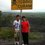# A pressing problem with Kinetic Energy. ALL PHYSICISTS HELP!!

the equation for kinetic energy is actually used as $\frac{1}{2}mv^{2}$ as it is a very useful quantity and actually is derived from the work done by a force, without any changes to internal energy or potential energy of the system.

$W = \int \Sigma\vec{F}\cdot d\vec{r}$

Subbing in $\Sigma\vec{F} = m\vec{a}$, you will get

$W = \int m\vec{a}\cdot d\vec{r}$

Subbing in $\vec{a} = \frac{d\vec{v}}{dt}$, you will get

$W = \int m\frac{d\vec{v}}{dt}\cdot d\vec{r}$

Since $\vec{v}=\frac{d\vec{r}}{dt}$, it implies that $d\vec{r} = \vec{v}dt$

$W = \int m\frac{d\vec{v}}{dt}\cdot\vec{v}dt$

$W = \int_{\vec{v}_i}^{\vec{v}_f} m\vec{v}\cdot d\vec{v}$

$W = \frac{1}{2}m(\vec{v}_f \cdot \vec{v}_f) - \frac{1}{2}m(\vec{v}_i\cdot\vec{v}_i) = \frac{1}{2}mv_f {}^{2} - \frac{1}{2}mv_i{}^{2}$

This showed that the quantity $\frac{1}{2}mv^{2}$ can be denoted as the energy of a moving object, and any change in kinetic energy must be from an external work done

However we know that the general definition of force is $\Sigma\vec{F}=\frac{d\vec{p}}{dt}$

Hence work would be $W = \int \frac{d\vec{p}}{dt}\cdot d\vec{r}$.

Then from that kinetic energy should be derived, as it more accurately depicts motion of an object of changing mass. Then how do we derive $\int \frac{d\vec{p}}{dt}\cdot d\vec{r}$6 years, 10 months ago

This discussion board is a place to discuss our Daily Challenges and the math and science related to those challenges. Explanations are more than just a solution — they should explain the steps and thinking strategies that you used to obtain the solution. Comments should further the discussion of math and science.

When posting on Brilliant:

• Use the emojis to react to an explanation, whether you're congratulating a job well done , or just really confused .
• Ask specific questions about the challenge or the steps in somebody's explanation. Well-posed questions can add a lot to the discussion, but posting "I don't understand!" doesn't help anyone.
• Try to contribute something new to the discussion, whether it is an extension, generalization or other idea related to the challenge.

MarkdownAppears as
*italics* or _italics_ italics
**bold** or __bold__ bold
- bulleted- list
• bulleted
• list
1. numbered2. list
1. numbered
2. list
Note: you must add a full line of space before and after lists for them to show up correctly
paragraph 1paragraph 2

paragraph 1

paragraph 2

[example link](https://brilliant.org)example link
> This is a quote
This is a quote
    # I indented these lines
# 4 spaces, and now they show
# up as a code block.

print "hello world"
# I indented these lines
# 4 spaces, and now they show
# up as a code block.

print "hello world"
MathAppears as
Remember to wrap math in $$ ... $$ or $ ... $ to ensure proper formatting.
2 \times 3 $2 \times 3$
2^{34} $2^{34}$
a_{i-1} $a_{i-1}$
\frac{2}{3} $\frac{2}{3}$
\sqrt{2} $\sqrt{2}$
\sum_{i=1}^3 $\sum_{i=1}^3$
\sin \theta $\sin \theta$
\boxed{123} $\boxed{123}$

Sort by:

It's similar. Hint: Product law for derivatives. $\frac{dp}{dt}=\frac{d(mv)}{dt}=m\cdot\frac{dv}{dt}+v\cdot\frac{dm}{dt}$. Note that $\frac{dm}{dt}=0$ for constant mass, that is the non-relativistic case. Note that $F=ma$ is not true for relativistic cases. (Sorry lazy to put vector arrows and stuff =P)

- 6 years, 10 months ago

But what if mass is not constant so $\frac{dm}{dt} \neq 0$

- 6 years, 10 months ago

Then you have to find out at what rate $m$ is changing wrt to $t$ and continue with the derivation.

- 6 years, 10 months ago

Would not that be equivalent to saying $KE\neq \frac{1}{2}mv^{2}$

- 6 years, 10 months ago

Exactly, that is what relativity is about.

- 6 years, 10 months ago

Actually according to relativity, our mass is increasing with velocity but not that much. So, the kinetic energy formula holds. Also, the formula is m_{real}=\frac{m_{rest}}{\sqrt{1-\frac{v^{2}}{c^{2}}}

- 6 years, 10 months ago

dmv/dt .dr here dr/dt =v and mdv will be left so we can procede in the same way above

- 6 years, 2 months ago